# Lecture 4

## Welcome!

• In previous weeks, we talked about images being made of smaller building blocks called pixels.
• Today, we will go into further detail about the zeros and ones that make up these images.
• Further, we will discuss how to access the underlying data stored in computer memory.

## Memory

• You can imagine a crime drama where an image is enhanced, enhanced, and enhanced, is not entirely real-life accurate. Indeed, if you keep zooming into an image, you will see pixels.• You can imagine as an image as a map of bits, where zeros represent black and ones represent white.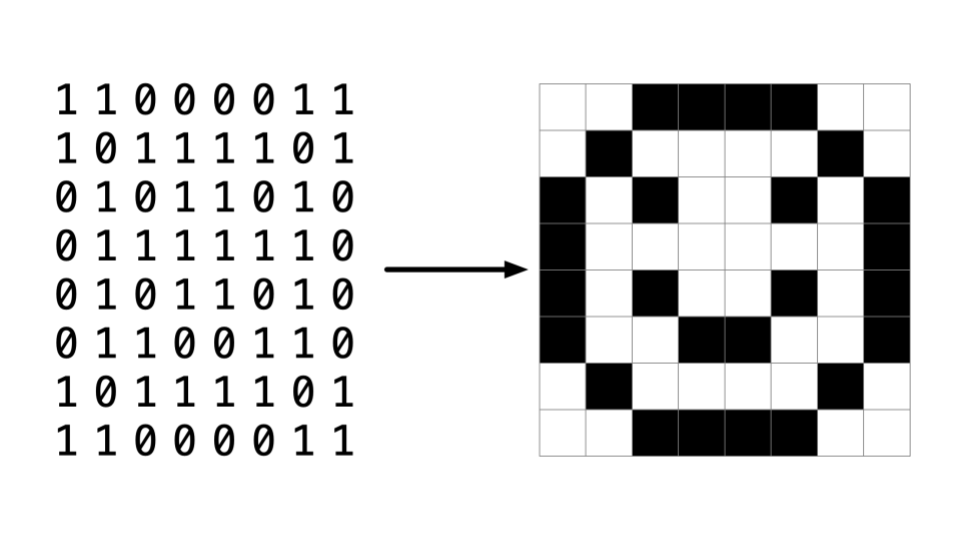• RGB, or red, green, blue, are numbers that represent the amount of each of these colors. In Adobe Photoshop, you can see these settings as follows: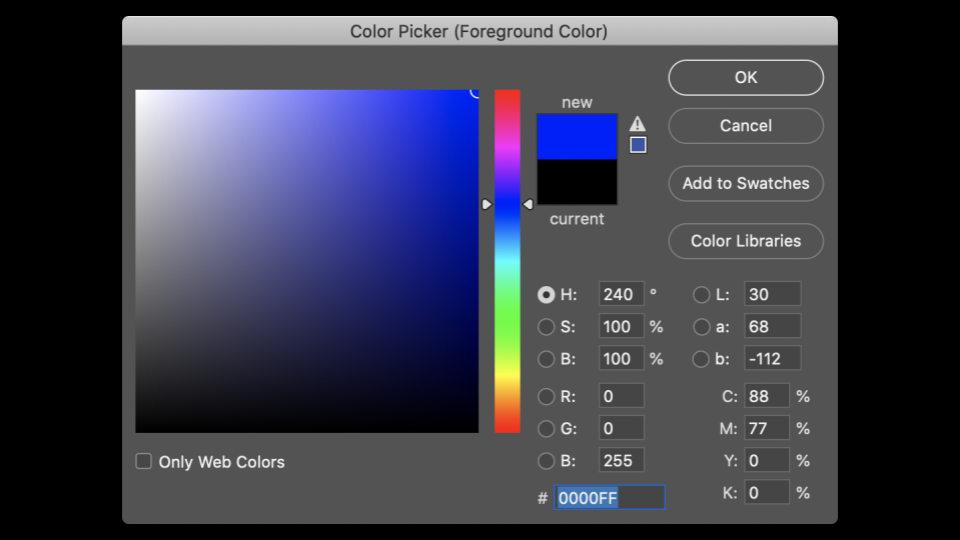Notice how the amount of red, blue, and green changes the color selected.

• You can see by the image above that color is not just represented in three values. At the bottom of the window, there is a special value made up of numbers and characters. 255 is represented as FF. Why might this be?

• Hexadecimal is a system of counting that has 16 counting values. They are as follows:

  0 1 2 3 4 5 6 7 8 9 a b c d e f


Notice that F represents 15.

• Hexadecimal is also known as base-16.
• When counting in hexadecimal, each column is a power of 16.
• The number 0 is represented as 00.
• The number 1 is represented as 01.
• The number 9 is represented by 09.
• The number 10 is represented as 0A.
• The number 15 is represented as 0F.
• The number 16 is represented as 10.
• The number 255 is represented as FF, because 16 x 15 (or F) is 240. Add 15 more to make 255. This is the highest number you can count using a two-digit hexadecimal system.
• Hexadecimal is useful because it can be represented using fewer digits. Hexadecimal allows us to represent information more succinctly.

• In weeks past, you may recall our artist rendering of concurrent blocks of memory. Applying hexadecimal numbering to each of these blocks of memory, you can visualize these as follows: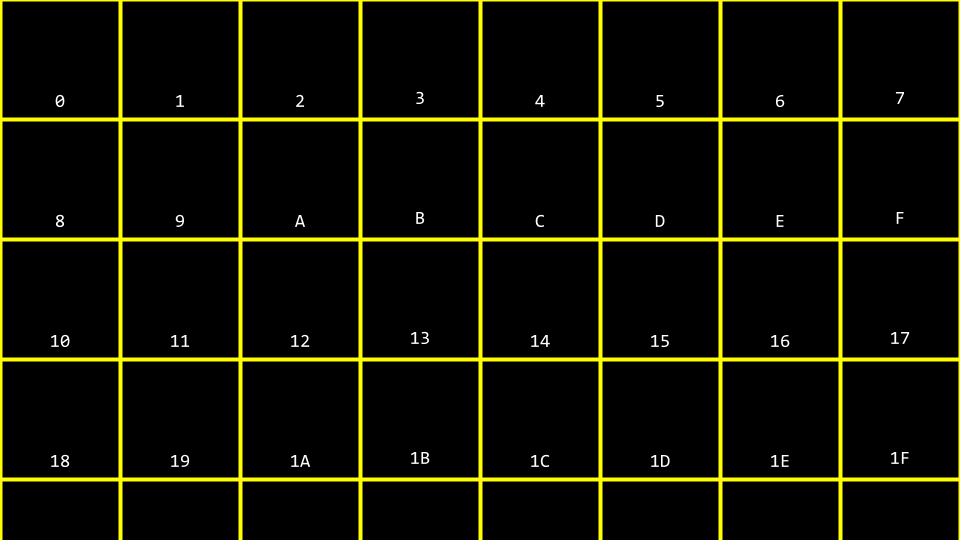• You can imagine how there may be confusion regarding whether the 10 block above may represent a location in memory or the value 10. Accordingly, by convention, all hexadecimal numbers are often represented with the 0x prefix as follows: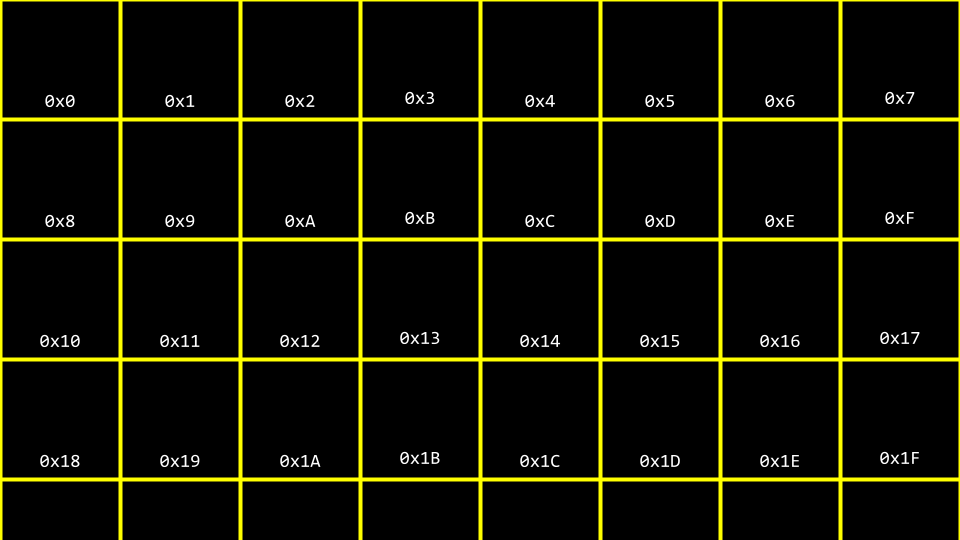• In your terminal window, type code addresses.c and write your code as follows:

#include <stdio.h>

int main(void)
{
int n = 50;
printf("%i\n", n);
}


Notice how n is stored in memory with the value 50.

• You can visualize how this program stores this value as follows: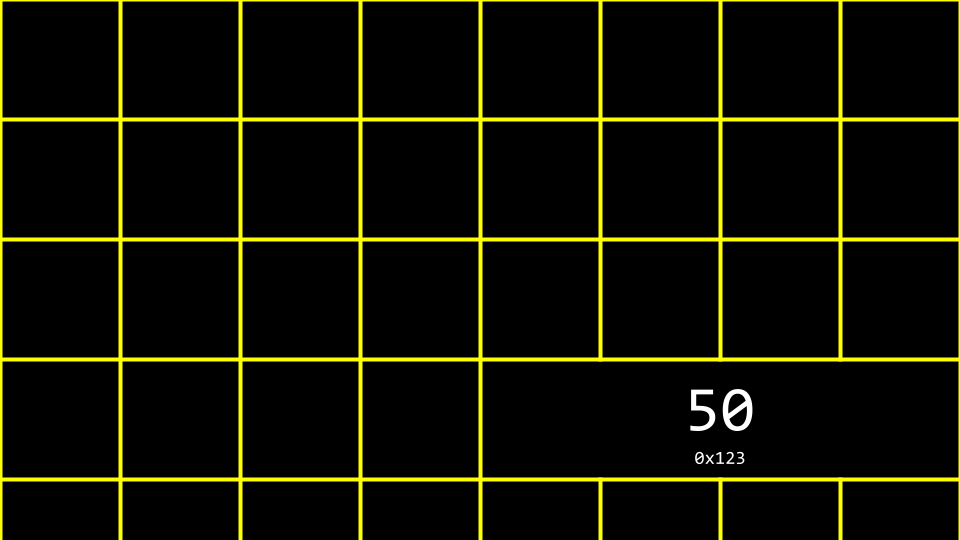• The C language has two powerful operators that relate to memory:

  & Provides the address of something stored in memory.
* Instructs the compiler to go to a location in memory.

• We can leverage this knowledge by modifying our code as follows:

#include <stdio.h>

int main(void)
{
int n = 50;
printf("%p\n", &n);
}


Notice the %p, which allows us to view the address of a location in memory. &n can be literally translated as “the address of n.” Executing this code will return an address of memory beginning with 0x.

## Pointers

• A pointer is a variable that contains the address of some value. Most succinctly, a pointer is an address in your computer’s memory.
• Consider the following code:

int n = 50;

int *p = &n;


Notice that p is a pointer that contains a number that is the address of an integer n.

• Modify your code as follows:

#include <stdio.h>

int main(void)
{
int n = 50;
int *p = &n;
printf("%p\n", p);
}


Notice that this code has the same effect as our previous code. We have simply leveraged our new knowledge of the & and * operators.

• You can visualize our code as follows: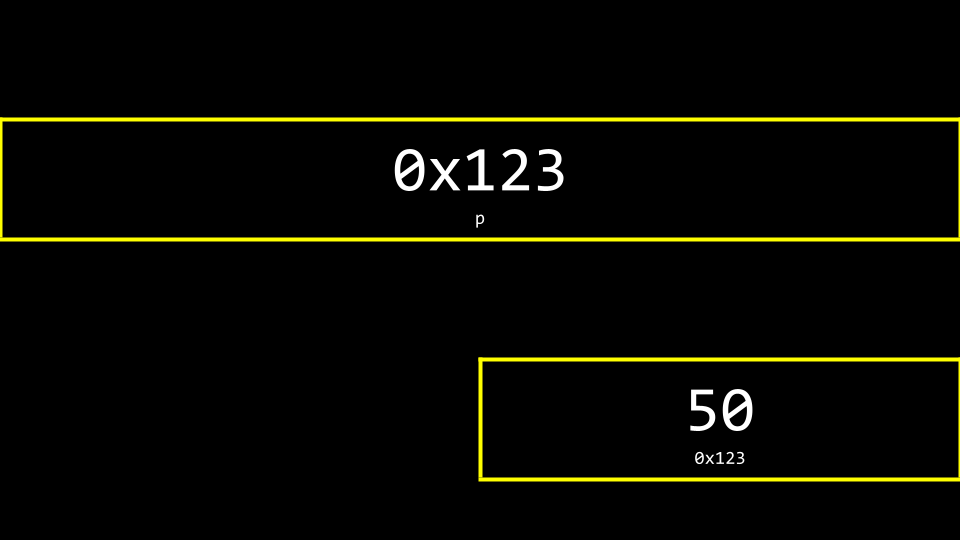Notice the pointer seems rather large. Indeed, a pointer is usually stored as an 8-byte value.

• You can more accurately visualize a pointer as one address that points to another: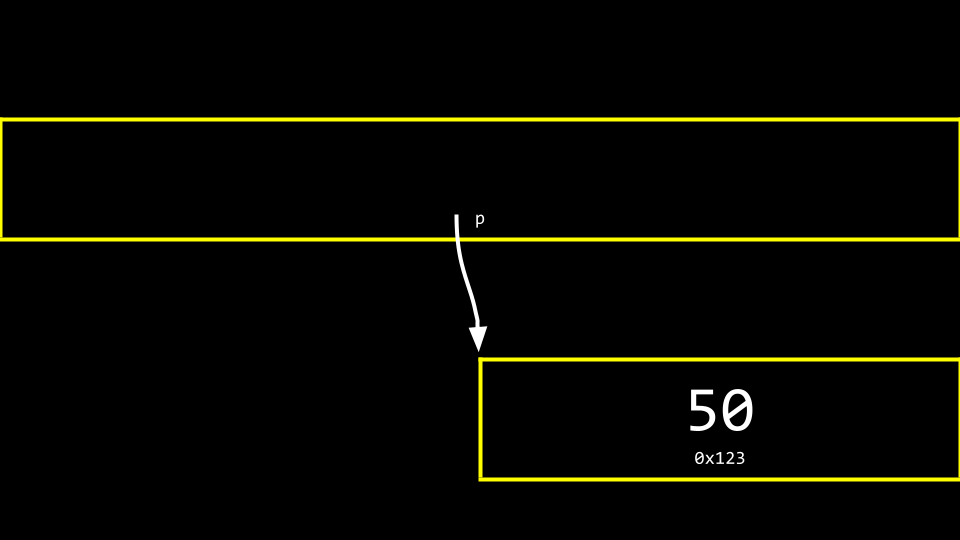• To illustrate the use of the * operator, consider the following:

#include <stdio.h>

int main(void)
{
int n = 50;
int *p = &n;
printf("%i\n", *p);
}


Notice that the printf line prints the integer at the location of p.

## Strings

• Now that we have a mental model for pointers, we can peel back a level of simplification that was offered earlier in this course.
• Recall that a string is simply an array of characters. For example, string s = "HI!" can be represented as follows: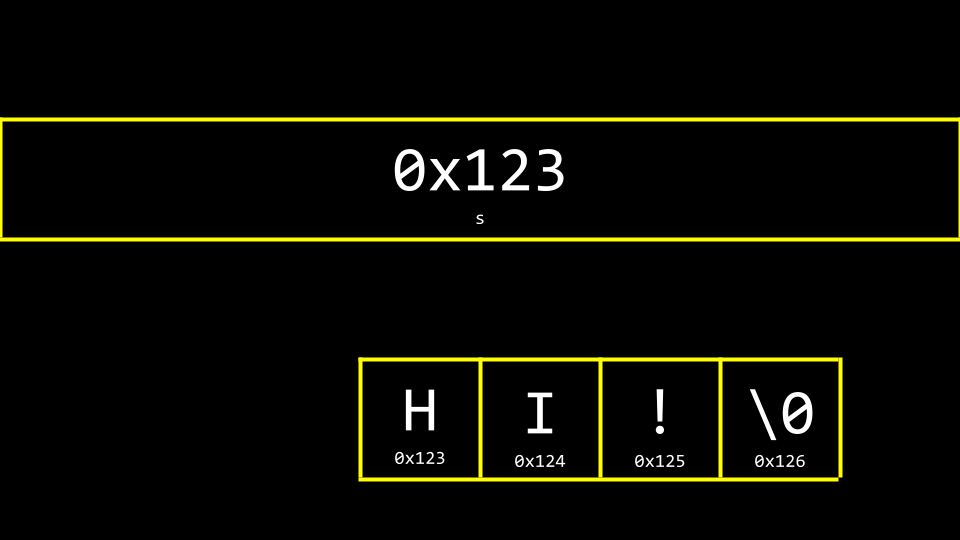• However, what is s really? Where is the s stored in memory? As you can imagine, s needs to be stored somewhere. You can visualize the relationship of s to the string as follows: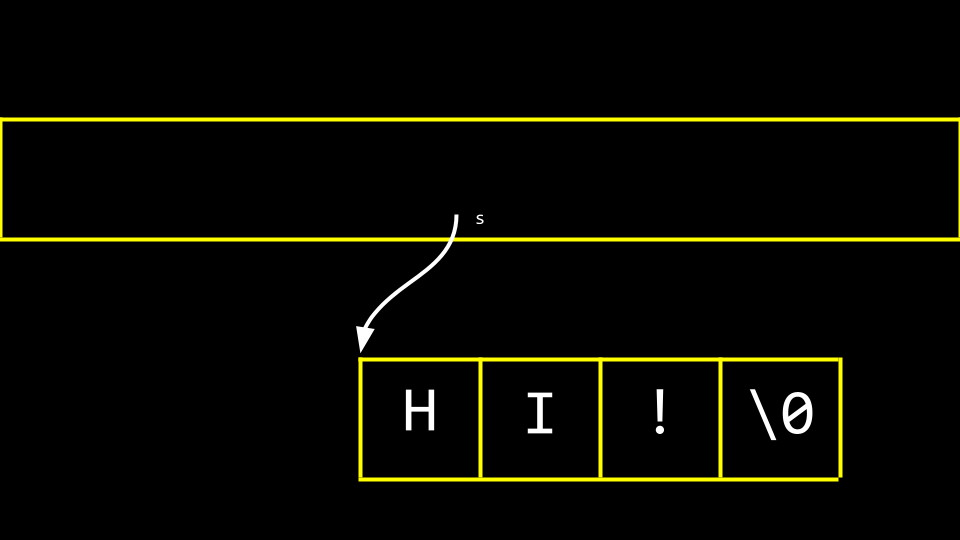Notice how a pointer called s tells the compiler where the first byte of the string exists in memory.

• Modify your code as follows:

#include <cs50.h>
#include <stdio.h>

int main(void)
{
string s = "HI!";
printf("%p\n", s);
printf("%p\n", &s);
printf("%p\n", &s);
printf("%p\n", &s);
printf("%p\n", &s);
}


Notice the above prints the memory locations of each character in the string s.

• Likewise, you can modify your code as follows:

#include <stdio.h>

int main(void)
{
char *s = "HI!";
printf("%s\n", s);
}


Notice that this code will present the string that starts at the location of s.

## Pointer Arithmetic

• You can modify your code to accomplish the same thing in a longer form as follows:

#include <stdio.h>

int main(void)
{
char *s = "HI!";
printf("%c\n", s);
printf("%c\n", s);
printf("%c\n", s);
}


Notice that we are printing each character at the location of s.

• Further, you can modify your code as follows:

#include <stdio.h>

int main(void)
{
char *s = "HI!";
printf("%c\n", *s);
printf("%c\n", *(s + 1));
printf("%c\n", *(s + 2));
}


Notice that the first character at the location of s is printed. Then, the character at the location s + 1 is printed, and so on.

• Can you imagine what would happen if you attempted to access something at location s + 50? Hackers sometimes attempt to gain access to items in memory they should not have access to. If you attempt this, the program will likely quit as a safety precaution.

## Comparing Strings

• A string of characters is simply an array of characters identified by its first byte.
• Recall that last week we proposed that we could not compare two strings using the == operator.
• Utilizing the == operator in an attempt to compare strings will attempt to compare the memory locations of the strings instead of the characters therein. Accordingly, we recommended the use of strcmp.
• To illustrate this, type code compare.c and write code as follows:

#include <cs50.h>
#include <stdio.h>

int main(void)
{
// Get two strings
char *s = get_string("s: ");
char *t = get_string("t: ");

if (s == t)
{
printf("Same\n");
}
else
{
printf("Different\n");
}
}


Noticing that typing in HI! for both strings still results in the output of Different.

• Why are these strings seemingly different? You can use the following to visualize why: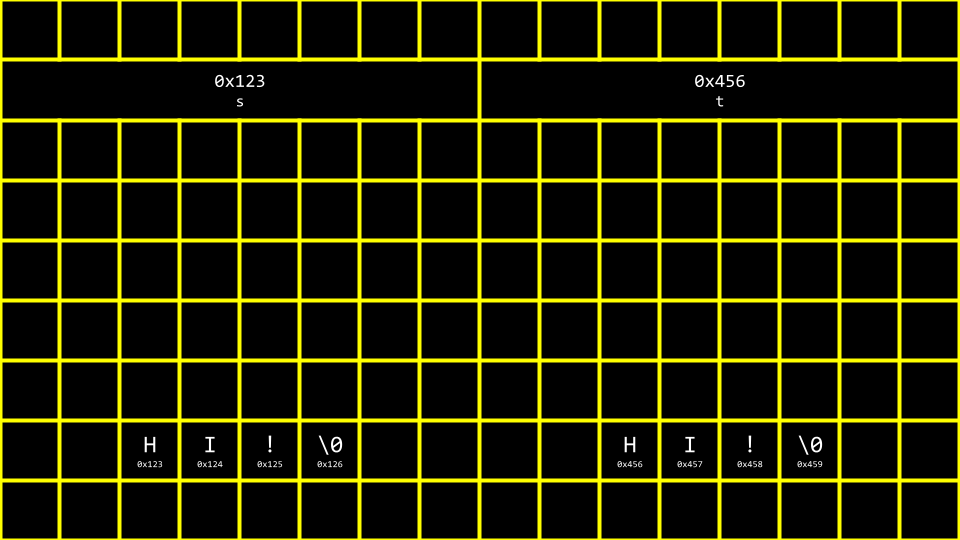• For clarity, you can see how the following image illustrates pointers pointing to two separate locations in memory: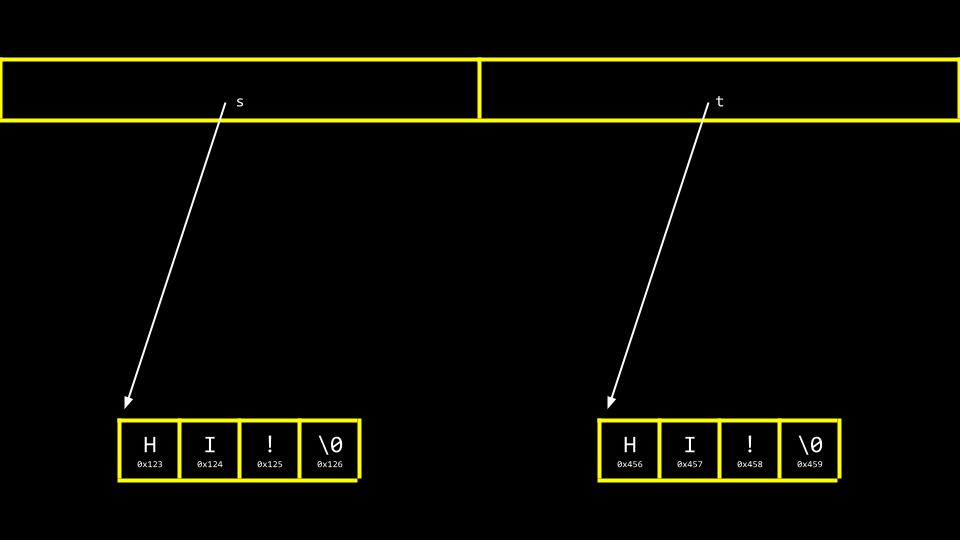• Modify your code as follows:

#include <cs50.h>
#include <stdio.h>

int main(void)
{
// Get two strings
char *s = get_string("s: ");
char *t = get_string("t: ");

// Print strings
printf("%s\n", s);
printf("%s\n", t);
}


Notice how we now have two separate strings stored likely at two separate locations.

• You can see the locations of these two stored strings with a small modification:

#include <cs50.h>
#include <stdio.h>

int main(void)
{
// Get two strings
char *s = get_string("s: ");
char *t = get_string("t: ");

printf("%p\n", s);
printf("%p\n", t);
}


Notice that the %s has been changed to %p in the print statement.

## Copying

• A common need in programming is to copy one string to another.
• In your terminal window, type code copy.c and write code as follows:

#include <cs50.h>
#include <ctype.h>
#include <stdio.h>
#include <string.h>

int main(void)
{
// Get a string
string s = get_string("s: ");

string t = s;

// Capitalize first letter in string
t = toupper(t);

// Print string twice
printf("s: %s\n", s);
printf("t: %s\n", t);
}


Notice that string t = s copies the address of s to t. This does not accomplish what we are desiring. The string is not copied – only the address is.

• Before we address this challenge, it’s important to ensure that we don’t experience a segmentation fault through our code, where we attempt to copy string s to string t, where string t does not exist. We can employ the strlen function as follows to assist with that:

#include <cs50.h>
#include <ctype.h>
#include <stdio.h>
#include <string.h>

int main(void)
{
// Get a string
string s = get_string("s: ");

string t = s;

// Capitalize first letter in string
if (strlen(t) > 0)
{
t = toupper(t);
}

// Print string twice
printf("s: %s\n", s);
printf("t: %s\n", t);
}


Notice that strlen is used to make sure string t exists. If it does not, nothing will be copied.

• You can visualize the above code as follows: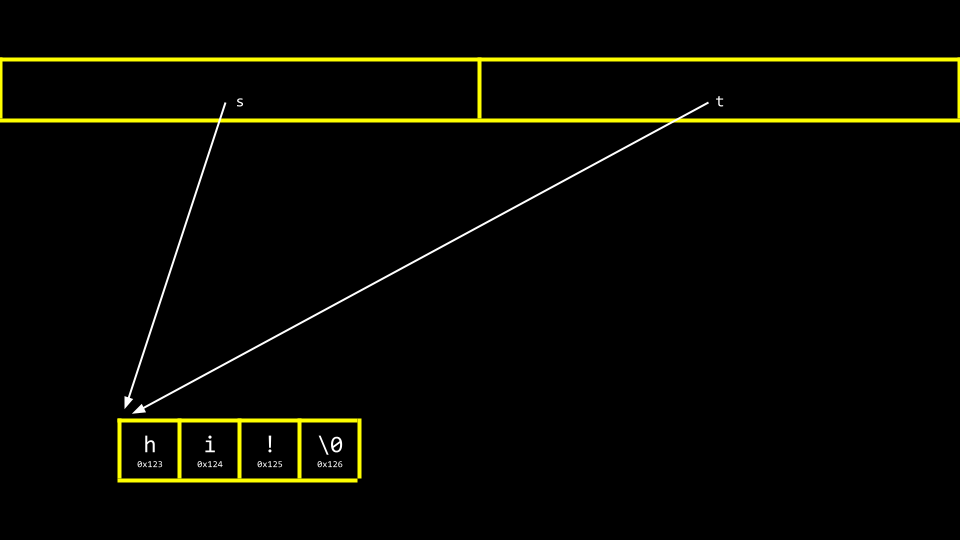Notice that s and t are still pointing at the same blocks of memory. This is not an authentic copy of a string. Instead, these are two pointers pointing at the same string.

• To be able to make an authentic copy of the string, we will need to introduce two new building blocks. First, malloc allows you, the programmer, to allocate a block of a specific size of memory. Second, free allows you to tell the compiler to free up that block of memory you previously allocated.

• We can modify our code to create an authentic copy of our string as follows:

#include <cs50.h>
#include <ctype.h>
#include <stdio.h>
#include <stdlib.h>
#include <string.h>

int main(void)
{
// Get a string
char *s = get_string("s: ");

// Allocate memory for another string
char *t = malloc(strlen(s) + 1);

// Copy string into memory, including '\0'
for (int i = 0; i <= strlen(s); i++)
{
t[i] = s[i];
}

// Capitalize copy
t = toupper(t);

// Print strings
printf("s: %s\n", s);
printf("t: %s\n", t);
}


Notice that malloc(strlen(s) + 1) creates a block of memory that is the length of the string s plus one. This allows for the inclusion of the null \0 character in our final, copied string. Then, the for loop walks through the string s and assigns each value to that same location on the string t.

• It turns out that there is an inefficiency in our code. Modify your code as follows:

#include <cs50.h>
#include <ctype.h>
#include <stdio.h>
#include <stdlib.h>
#include <string.h>

int main(void)
{
// Get a string
char *s = get_string("s: ");

// Allocate memory for another string
char *t = malloc(strlen(s) + 1);

// Copy string into memory, including '\0'
for (int i = 0, n = strlen(s); i <= n; i++)
{
t[i] = s[i];
}

// Capitalize copy
t = toupper(t);

// Print strings
printf("s: %s\n", s);
printf("t: %s\n", t);
}


Notice that n = strlen(s) is defined now in the left-hand side of the for loop. It’s best not to call unneeded functions in the middle condition of the for loop, as it will run over and over again. When moving n = strlen(s) to the left-hand side, the function strlen only runs once.

• The C Language has a built-in function to copy strings called strcpy. It can be implemented as follows:

#include <cs50.h>
#include <ctype.h>
#include <stdio.h>
#include <stdlib.h>
#include <string.h>

int main(void)
{
// Get a string
char *s = get_string("s: ");

// Allocate memory for another string
char *t = malloc(strlen(s) + 1);

// Copy string into memory
strcpy(t, s);

// Capitalize copy
t = toupper(t);

// Print strings
printf("s: %s\n", s);
printf("t: %s\n", t);
}


Notice that strcpy does the same work that our for loop previously did.

• Both get_string and malloc return NULL, a special value in memory, in the event that something goes wrong. You can write code that can check for this NULL condition as follows:

#include <cs50.h>
#include <ctype.h>
#include <stdio.h>
#include <stdlib.h>
#include <string.h>

int main(void)
{
// Get a string
char *s = get_string("s: ");
if (s == NULL)
{
return 1;
}

// Allocate memory for another string
char *t = malloc(strlen(s) + 1);
if (t == NULL)
{
return 1;
}

// Copy string into memory
strcpy(t, s);

// Capitalize copy
if (strlen(t) > 0)
{
t = toupper(t);
}

// Print strings
printf("s: %s\n", s);
printf("t: %s\n", t);

// Free memory
free(t);
return 0;
}


Notice that if the string obtained is of length 0 or malloc fails, NULL is returned. Further, notice that free lets the computer know you are done with this block of memory you created via malloc.

## Valgrind

• Valgrind is a tool that can check to see if there are memory-related issues with your programs wherein you utilized malloc. Specifically, it checks to see if you free all the memory you allocated.
• Consider the following code:

#include <stdio.h>
#include <stdlib.h>

int main(void)
{
int *x = malloc(3 * sizeof(int));
x = 72;
x = 73;
x = 33;
}


Notice that running this program does not cause any errors. While malloc is used to allocate enough memory for an array, the code fails to free that allocated memory.

• If you type make memory followed by valgrind ./memory, you will get a report from valgrind that will report where memory has been lost as a result of your program.
• You can modify your code as follows:

#include <stdio.h>
#include <stdlib.h>

int main(void)
{
int *x = malloc(3 * sizeof(int));
x = 72;
x = 73;
x = 33;
free(x);
}


Notice that running valgrind again now results in now memory leaks.

## Garbage Values

• When you ask the compiler for a block of memory, there is no guarantee that this memory will be empty.
• It’s very possible that this memory that you allocated was previously utilized by the computer. Accordingly, you may see junk or garbage values. This is a result of you getting a block of memory but not initializing it. For example, consider the following code:

#include <stdio.h>
#include <stdlib.h>

int main(void)
{
int scores;
for (int i = 0; i < 1024; i++)
{
printf("%i\n", scores[i]);
}
}


Notice that running this code will allocate 1024 locations in memory for your array, but the for loop will likely show that not all values therein are 0. It’s always best practice to be aware of the potential for garbage values when you do not initialize blocks of memory to some other value like zero or otherwise.

## Swap

• In the real world, a common need in programming is to swap two values. Naturally, it’s hard to swap two variables without a temporary holding space. In practice, you can type code swap.c and write code as follows to see this in action:

#include <stdio.h>

void swap(int a, int b);

int main(void)
{
int x = 1;
int y = 2;

printf("x is %i, y is %i\n", x, y);
swap(x, y);
printf("x is %i, y is %i\n", x, y);
}

void swap(int a, int b)
{
int tmp = a;
a = b;
b = tmp;
}


Notice that while this code runs, it does not work. The values, even after being sent to the swap function, do not swap. Why?

• When you pass values to a function, you are only providing copies. In previous weeks, we discussed the concept of scope. The values of x and y created in the curly {} braces of the main function only have the scope of the main function. Consider the following image: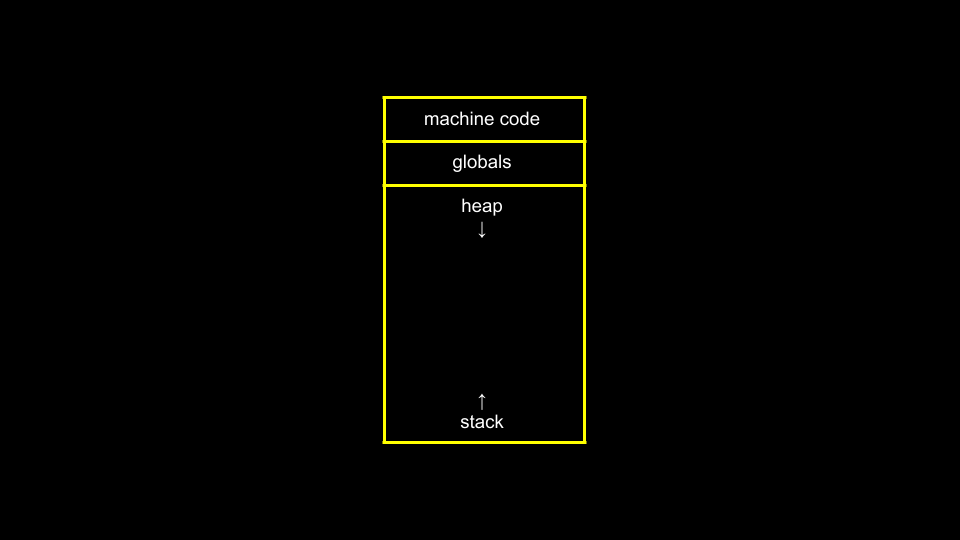Notice that global variables, which we have not used in this course, live in one place in memory. Various functions are stored in the stack in another area of memory.

• Now, consider the following image: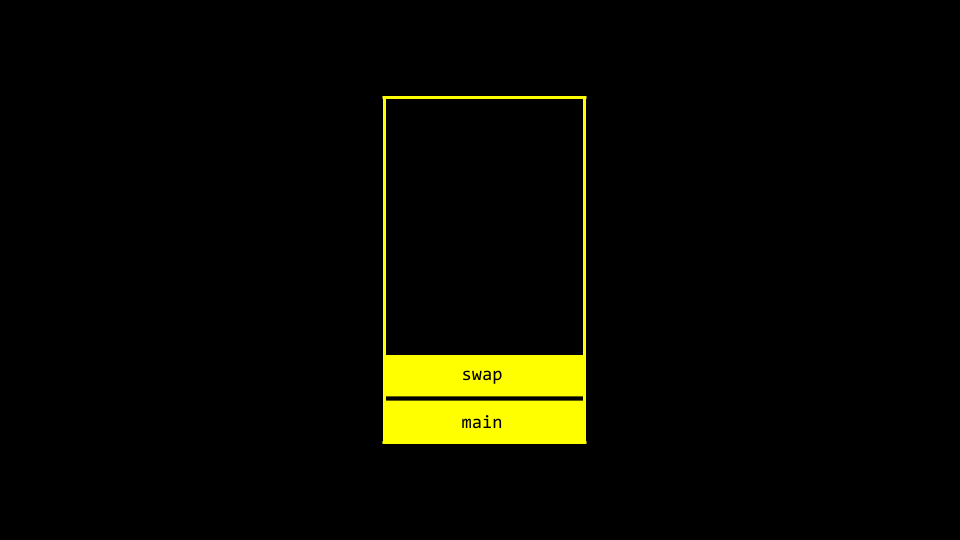Notice that main and swap have two separate frames or areas of memory. Therefore, we cannot simply pass the values from one function to another to change them.

• Modify your code as follows:

#include <stdio.h>

void swap(int *a, int *b);

int main(void)
{
int x = 1;
int y = 2;

printf("x is %i, y is %i\n", x, y);
swap(&x, &y);
printf("x is %i, y is %i\n", x, y);
}

void swap(int *a, int *b)
{
int tmp = *a;
*a = *b;
*b = tmp;
}


Notice that variables are not passed by value but by reference. That is, the addresses of a and b are provided to the function. Therefore, the swap function can know where to make changes to the actual a and b from the main function.

• You can visualize this as follows: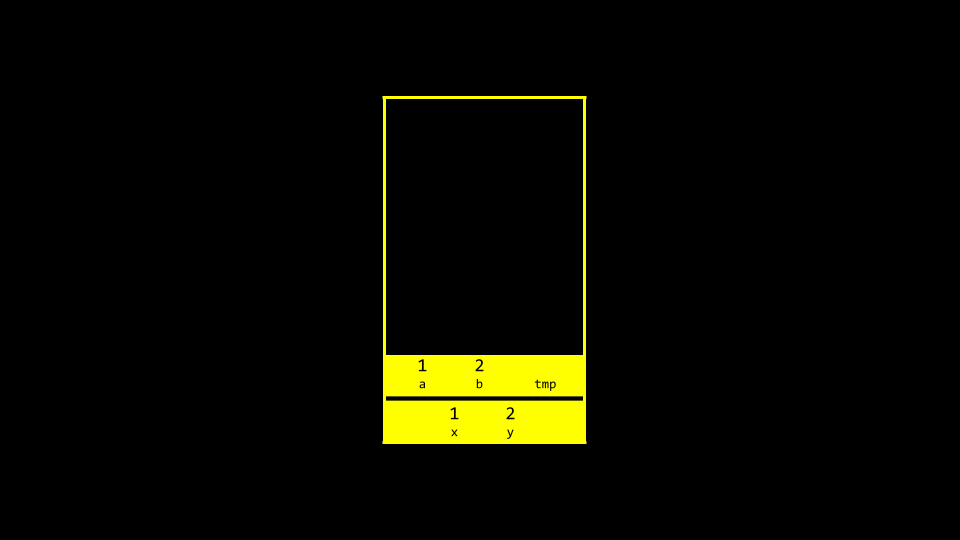## Overflow

• A heap overflow is when you overflow the heap, touching areas of memory you are not supposed to.
• A stack overflow is when too many functions are called, overflowing the amount of memory available.
• Both of these are considered buffer overflows.

## scanf

• In CS50, we have created functions like get_int to simplify the act of getting input from the user.
• scanf is a built-in function that can get user input.
• We can reimplement get_int rather easily using scanf as follows:

#include <stdio.h>

int main(void)
{
int x;
printf("x: ");
scanf("%i", &x);
printf("x: %i\n", x);
}


Notice that the value of x is stored at the location of x in the line scanf("%i", &x).

• However, attempting to reimplement get_string is not easy. Consider the following:

#include <stdio.h>

int main(void)
{
char *s;
printf("s: ");
scanf("%s", s);
printf("s: %s\n", s);
}


Notice that no & is required because strings are special. Still, this program will not function. Nowhere in this program do we allocate the amount of memory required for our string.

• We can modify our code as follows:

#include <stdio.h>

int main(void)
{
char s;
printf("s: ");
scanf("%s", s);
printf("s: %s\n", s);
}


Notice that if we pre-allocate an array of size 4, we can type cat and the program functions. However, a string larger than this would create an error.

## Files

• You can read from and manipulate files. While this topic will be discussed further in a future week, consider the following code for phonebook.c:

#include <cs50.h>
#include <stdio.h>
#include <string.h>

int main(void)
{
// Open CSV file
FILE *file = fopen("phonebook.csv", "a");

// Get name and number
char *name = get_string("Name: ");
char *number = get_string("Number: ");

// Print to file
fprintf(file, "%s,%s\n", name, number);

// Close file
fclose(file);
}


Notice that this code uses pointers to access the file.

• You can create a file called phonebook.csv in advance of running the above code. After running the above program and inputting a name and phone number, you will notice that this data persists in your CSV file.

## Summing Up

In this lesson, you learned about pointers that provide you with the ability to access and manipulate data at specific memory locations. Specifically, we delved into…

• Memory
• scanf